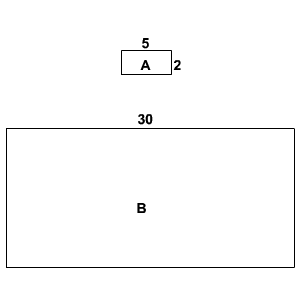# Scale Drawings

Suppose you need to draw a model of your school compound that will proportionally show all the landmarks. How will you do that?

You will need to fix a scale factor and find the proportional distances between the landmarks.

That is, if the playground is $\text{500}\text{\hspace{0.17em}}\text{\hspace{0.17em}}\text{m}$ away from the music room and the scale is $1\text{\hspace{0.17em}}\text{\hspace{0.17em}}\text{cm}:100\text{\hspace{0.17em}}\text{\hspace{0.17em}}\text{m}$ , then in the scale drawing, the two landmarks will be $\text{5}\text{\hspace{0.17em}}\text{\hspace{0.17em}}\text{cm}$ away.

You can use the distance between any two points in the scale drawing to find the actual distance between them.

Blueprints and maps are examples of scale drawings of objects. The scale factor of the drawing will be the ratio of the size of an object in the drawing to its actual size.

Any pair of similar geometrical figures has a scale factor that can be used to find the perimeter or the area . The ratio of the perimeters will be the same as the scale factor; the ratio of the areas will be the square of the scale factor.

Example 1:

Chris is making a scale drawing of a nearby zoo with a scale of $1\text{\hspace{0.17em}}\text{\hspace{0.17em}}\text{cm}:400\text{\hspace{0.17em}}\text{\hspace{0.17em}}\text{m}$ . If the lions' cage and the snake-park are apart in the scale drawing, what is the actual distance the two places?

The corresponding lengths of the scale drawing and the actual place will be proportional. That is, if $x$ is the actual distance between the two places then,

$1\text{\hspace{0.17em}}\text{\hspace{0.17em}}\text{cm}:400\text{\hspace{0.17em}}\text{\hspace{0.17em}}\text{m}=6\text{\hspace{0.17em}}\text{\hspace{0.17em}}\text{cm}:x\text{\hspace{0.17em}}\text{\hspace{0.17em}}\text{m}$ .

Write this as a proportion equation.

$\frac{1\text{\hspace{0.17em}}\text{\hspace{0.17em}}\text{cm}}{400\text{\hspace{0.17em}}\text{\hspace{0.17em}}\text{m}}=\frac{6\text{\hspace{0.17em}}\text{\hspace{0.17em}}\text{cm}}{x\text{\hspace{0.17em}}\text{\hspace{0.17em}}\text{m}}$

Cross multiply and cancel units.

$\begin{array}{l}1\cdot x=6\cdot 400\\ x=2400\end{array}$

Therefore, the lions' cage and the snake-park are $2400$ m apart.

Example 2:

The length of a rectangular microchip is $2$ mm whereas an enlarged scale drawing of the same has a length of $6$ cm. What is the scale factor?

To compare the sizes, convert the lengths into the same unit.

$1\text{\hspace{0.17em}}\text{\hspace{0.17em}}\text{cm}=10\text{\hspace{0.17em}}\text{\hspace{0.17em}}\text{mm}$

So, $6\text{\hspace{0.17em}}\text{\hspace{0.17em}}\text{cm}=\left(6×10\right)\text{\hspace{0.17em}}\text{\hspace{0.17em}}\text{mm}$ .

That is, the length in the scale drawing is $60$ mm.

Then the scale factor is $\frac{60\text{\hspace{0.17em}}\text{\hspace{0.17em}}\text{mm}}{2\text{\hspace{0.17em}}\text{\hspace{0.17em}}\text{mm}}=30$ . (That is, the ratio $30:1$ ).

Example 3:

Consider the rectangles shown.What is the perimeter of the rectangle $\text{B}$ ?

Consider the ratio of the corresponding sides of the two rectangles. The side of length $5$ of rectangle $\text{A}$ corresponds to the side of length $30$ in rectangle $\text{B}$ .

So, the scale factor is $30:5$ , or $\frac{30}{5}=6$ .

The ratio of the perimeters is the same as the ratio of corresponding sides.

The perimeter of the rectangle $\text{A}$ is $2\left(2\right)+2\left(5\right)=14$ units, and the scale factor is $6$ .

Therefore, the perimeter of the rectangle $\text{B}$ is $6×14=84$ units.

Example 4:

As a part of her school project, Angelina made a scale drawing of her science lab using a scale of $1\text{\hspace{0.17em}}\text{\hspace{0.17em}}\text{cm}:10\text{\hspace{0.17em}}\text{\hspace{0.17em}}\text{m}$ . Since the model was too small she was asked to double the size. What will be the new scale factor?

To double the size of the scale drawing, the scale should be halved. (Because we are shrinking the lab half as much.) That is, the new scale should be $1\text{\hspace{0.17em}}\text{\hspace{0.17em}}\text{cm}:5\text{\hspace{0.17em}}\text{\hspace{0.17em}}\text{m}$ .